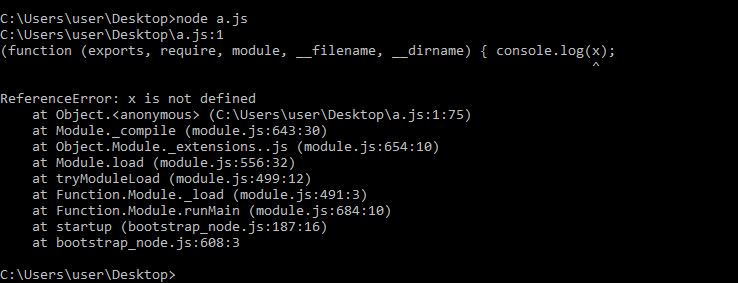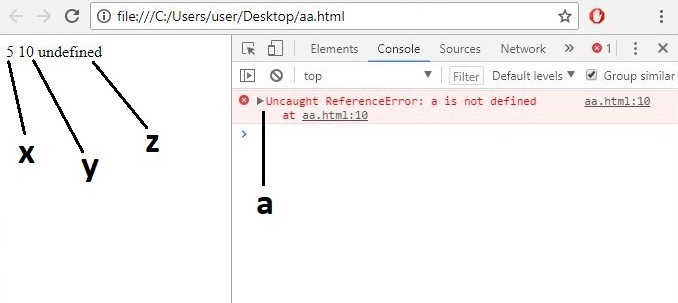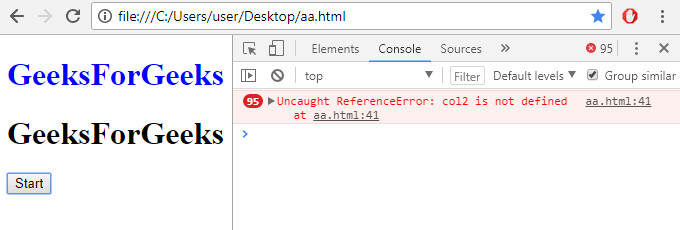# Difference between var and let in JavaScript

• Difficulty Level : Basic
• Last Updated : 04 Jul, 2022

var and let are both used for variable declaration in javascript but the difference between them is that var is function scoped and let is block scoped. It can be said that a variable declared with var is defined throughout the program as compared to let. An example will clarify the difference even better

Example of var:

```Input:
console.log(x);
var x=5;
console.log(x);
Output:
undefined
5```Example of let:

```Input:
console.log(x);
let x=5;
console.log(x);
Output:
Error```Let’s see code in JavaScript: Code #1:

## HTML

 `<``html``>` `<``body``>``    ``<``script``>``        ``// calling x after definition``        ``var x = 5;``        ``document.write(x, "\n");` `        ``// calling y after definition``        ``let y = 10;``        ``document.write(y, "\n");` `        ``// calling var z before definition will return undefined``        ``document.write(z, "\n");``        ``var z = 2;` `        ``// calling let a before definition will give error``        ``document.write(a);``        ``let a = 3;``    ````` `                           `

### OutputCode #2: In the following code, clicking start will call a function that changes the color of the two headings every 0.5sec. The color of first heading is stored in a var and the second one is declared by using let. Both of them are then accessed outside the function block. Var will work but the variable declared using let will show an error because let is block scoped.

## HTML

 ```<``html``>` `<``head``>``    ``<``title``>Var vs Let``` `<``body``>` `    ``<``h1` `id``=``"var"` `style``=``"color:black;"``>GeeksForGeeks``    ``<``h1` `id``=``"let"` `style``=``"color:black;"``>GeeksForGeeks``    ``<``button` `id``=``"btn"` `onclick``=``"colour()"``>Start``    ``` `    ``<``script` `type``=``"text/javascript"``>``        ``function colour() {` `            ``setInterval(function() {` `                ``if (document.getElementById('var').style.color == 'black')``                    ``var col1 = 'blue';``                ``else``                    ``col1 = 'black';` `                ``// setting value of color 1 through var` `                ``if (document.getElementById('let').style.color == 'black') {``                    ``let col2 = 'red';``                ``} else {``                    ``col2 = 'black';``                ``}` `                ``// setting value of color 2 through let` `                ``document.getElementById('var').style.color = col1;` `                ``document.getElementById('let').style.color = col2;` `                ``// changing color of h1 in html``            ``}, 500);` `        ``}``    ````` ``

Output:JavaScript is best known for web page development but it is also used in a variety of non-browser environments. You can learn JavaScript from the ground up by following this JavaScript Tutorial and JavaScript Examples.

Let us understand the differences in a tabular form -:

My Personal Notes arrow_drop_up